### TWOSAMPLEFREQ Statement

TWOSAMPLEFREQ <options> ;

The TWOSAMPLEFREQ statement performs power and sample size analyses for tests of two independent proportions. Pearson’s chi-square, Fisher’s exact, and likelihood ratio chi-square tests are supported.

#### Summary of Options

Table 71.19 summarizes the options available in the TWOSAMPLEFREQ statement.

Table 71.19: TWOSAMPLEFREQ Statement Options

Option

Description

Define analysis

Specifies the statistical analysis

Specify analysis information

Specifies the significance level

Specifies the null odds ratio

Specifies the null proportion difference

Specifies the null relative risk

Specifies the number of sides and the direction of the statistical test or confidence interval

Specify effects

Specifies the two independent proportions,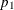and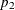Specifies the odds ratio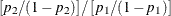Specifies the proportion difference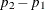Specifies the reference proportionSpecifies the relative risk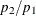Specify sample size and allocation

Specifies the two group sample sizes

Specifies the sample size allocation weights for the two groups

Enables fractional input and output for sample sizes

Specifies the common sample size per group

Specifies the sample size

Specify power

Specifies the desired power of the test

Control ordering in output

Controls the output order of parameters

Table 71.20 summarizes the valid result parameters for different analyses in the TWOSAMPLEFREQ statement.

Table 71.20: Summary of Result Parameters in the TWOSAMPLEFREQ Statement

Analyses

Solve For

Syntax

TEST=FISHER

Power

Sample size

TEST=LRCHI

Power

Sample size

TEST=PCHI

Power

Sample size

#### Dictionary of Options

ALPHA=number-list

specifies the level of significance of the statistical test. The default is 0.05, corresponding to the usual 0.05100% = 5% level of significance. See the section Specifying Value Lists in Analysis Statements for information about specifying the number-list.

GROUPPROPORTIONS=grouped-number-list
GPROPORTIONS=grouped-number-list
GROUPPS=grouped-number-list
GPS=grouped-number-list

specifies the two independent proportions,and. See the section Specifying Value Lists in Analysis Statements for information about specifying the grouped-number-list.

GROUPNS=grouped-number-list
GNS=grouped-number-list

specifies the two group sample sizes. See the section Specifying Value Lists in Analysis Statements for information about specifying the grouped-number-list.

GROUPWEIGHTS=grouped-number-list
GWEIGHTS=grouped-number-list

specifies the sample size allocation weights for the two groups. This option controls how the total sample size is divided between the two groups. Each pair of values for the two groups represents relative allocation weights. Additionally, if the NFRACTIONAL option is not used, the total sample size is restricted to be equal to a multiple of the sum of the two group weights (so that the resulting design has an integer sample size for each group while adhering exactly to the group allocation weights). Values must be integers unless the NFRACTIONAL option is used. The default value is (1 1), a balanced design with a weight of 1 for each group. See the section Specifying Value Lists in Analysis Statements for information about specifying the grouped-number-list.

NFRACTIONAL
NFRAC

enables fractional input and output for sample sizes. See the section Sample Size Adjustment Options for information about the ramifications of the presence (and absence) of the NFRACTIONAL option.

NPERGROUP=number-list
NPERG=number-list

specifies the common sample size per group or requests a solution for the common sample size per group with a missing value (NPERGROUP=.). Use of this option implicitly specifies a balanced design. See the section Specifying Value Lists in Analysis Statements for information about specifying the number-list.

NTOTAL=number-list

specifies the sample size or requests a solution for the sample size with a missing value (NTOTAL=.). See the section Specifying Value Lists in Analysis Statements for information about specifying the number-list.

NULLODDSRATIO=number-list
NULLOR=number-list

specifies the null odds ratio. The default value is 1. This option can be used only with the ODDSRATIO= option in the TEST=PCHI analysis. See the section Specifying Value Lists in Analysis Statements for information about specifying the number-list.

NULLPROPORTIONDIFF=number-list
NULLPDIFF=number-list

specifies the null proportion difference. The default value is 0. This option can be used only with the GROUPPROPORTIONS= or PROPORTIONDIFF= option in the TEST=PCHI analysis. See the section Specifying Value Lists in Analysis Statements for information about specifying the number-list.

NULLRELATIVERISK=number-list
NULLRR=number-list

specifies the null relative risk. The default value is 1. This option can be used only with the RELATIVERISK= option in the TEST=PCHI analysis. See the section Specifying Value Lists in Analysis Statements for information about specifying the number-list.

ODDSRATIO=number-list
OR=number-list

specifies the odds ratio. See the section Specifying Value Lists in Analysis Statements for information about specifying the number-list.

OUTPUTORDER=INTERNAL
OUTPUTORDER=REVERSE
OUTPUTORDER=SYNTAX

controls how the input and default analysis parameters are ordered in the output. OUTPUTORDER=INTERNAL (the default) arranges the parameters in the output according to the following order of their corresponding options:

The OUTPUTORDER=SYNTAX option arranges the parameters in the output in the same order in which their corresponding options are specified in the TWOSAMPLEFREQ statement. The OUTPUTORDER=REVERSE option arranges the parameters in the output in the reverse of the order in which their corresponding options are specified in the TWOSAMPLEFREQ statement.

POWER=number-list

specifies the desired power of the test or requests a solution for the power with a missing value (POWER=.). The power is expressed as a probability, a number between 0 and 1, rather than as a percentage. See the section Specifying Value Lists in Analysis Statements for information about specifying the number-list.

PROPORTIONDIFF=number-list
PDIFF=number-list

specifies the proportion difference. See the section Specifying Value Lists in Analysis Statements for information about specifying the number-list.

REFPROPORTION=number-list
REFP=number-list

specifies the reference proportion. See the section Specifying Value Lists in Analysis Statements for information about specifying the number-list.

RELATIVERISK=number-list
RR=number-list

specifies the relative risk. See the section Specifying Value Lists in Analysis Statements for information about specifying the number-list.

SIDES=keyword-list

specifies the number of sides (or tails) and the direction of the statistical test or confidence interval. See the section Specifying Value Lists in Analysis Statements for information about specifying the keyword-list. Valid keywords and their interpretation are as follows:

1

one-sided with alternative hypothesis in same direction as effect

2

two-sided

U

upper one-sided with alternative greater than null value

L

lower one-sided with alternative less than null value

If the effect size is zero, then SIDES=1 is not permitted; instead, specify the direction of the test explicitly in this case with either SIDES=L or SIDES=U. The default value is 2.

TEST=FISHER
TEST=LRCHI
TEST=PCHI

specifies the statistical analysis. TEST=FISHER specifies Fisher’s exact test. TEST=LRCHI specifies the likelihood ratio chi-square test. TEST=PCHI (the default) specifies Pearson’s chi-square test.

#### Restrictions on Option Combinations

To specify the proportions, choose one of the following parameterizations:

To specify the sample size and allocation, choose one of the following parameterizations:

• sample size per group in a balanced design (by using the NPERGROUP= option)

• total sample size and allocation weights (by using the NTOTAL= and GROUPWEIGHTS= options)

• individual group sample sizes (by using the GROUPNS= option)

#### Option Groups for Common Analyses

This section summarizes the syntax for the common analyses supported in the TWOSAMPLEFREQ statement.

##### Pearson Chi-Square Test for Two Proportions

You can use the NPERGROUP= option in a balanced design and express effects in terms of the individual proportions, as in the following statements. Default values for the SIDES= and ALPHA= options specify a two-sided test with a significance level of 0.05.

proc power;
twosamplefreq test=pchi
groupproportions = (.15 .25)
nullproportiondiff = .03
npergroup = 50
power = .;
run;


You can also specify an unbalanced design by using the NTOTAL= and GROUPWEIGHTS= options and express effects in terms of the odds ratio. The default value of the NULLODDSRATIO= option specifies a test of no effect.

proc power;
twosamplefreq test=pchi
oddsratio = 2.5
refproportion = 0.3
groupweights = (1 2)
ntotal = .
power = 0.8;
run;


You can also specify sample sizes with the GROUPNS= option and express effects in terms of relative risks. The default value of the NULLRELATIVERISK= option specifies a test of no effect.

proc power;
twosamplefreq test=pchi
relativerisk = 1.5
refproportion = 0.2
groupns = 40 | 60
power = .;
run;


You can also express effects in terms of the proportion difference. The default value of the NULLPROPORTIONDIFF= option specifies a test of no effect, and the default value of the GROUPWEIGHTS= option specifies a balanced design.

proc power;
twosamplefreq test=pchi
proportiondiff = 0.15
refproportion = 0.4
ntotal = 100
power = .;
run;

##### Fisher’s Exact Conditional Test for Two Proportions

The following statements demonstrate a power computation for Fisher’s exact conditional test for two proportions. Default values for the SIDES= and ALPHA= options specify a two-sided test with a significance level of 0.05.

proc power;
twosamplefreq test=fisher
groupproportions = (.35 .15)
npergroup = 50
power = .;
run;

##### Likelihood Ratio Chi-Square Test for Two Proportions

The following statements demonstrate a sample size computation for the likelihood ratio chi-square test for two proportions. Default values for the SIDES= and ALPHA= options specify a two-sided test with a significance level of 0.05.

proc power;
twosamplefreq test=lrchi
oddsratio = 2
refproportion = 0.4
npergroup = .
power = 0.9;
run;